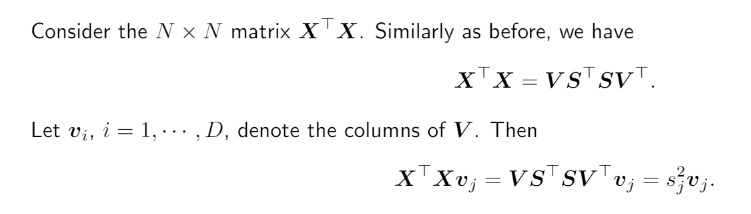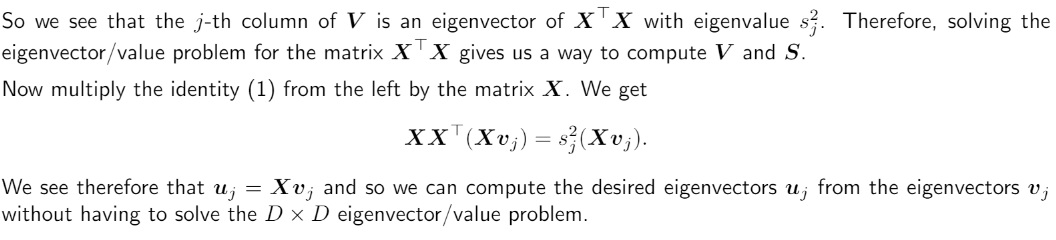### Lab 12 Problem 1

Hi,

1) Could you please give me more details on how we obtain this result : (edit : figured it out)I think that considering that V is orthogonal, V^T @ v_j should be equal to a vector where v_j = 1 else 0 because v_i @ v_j = 0 if i != j else 1.

Thus, I come up with a different result.

2) I also don't understand the second part of the solution.We have a way to compute efficiently s_j² but we are losing the sign of s_j. Is it important ?

Also, I don't get what is u_j and how we obtain the equality u_j = X_vj. Is it a column or a row of U ?

My first guess is that :
X^T @ X @ v_j = s_j² v_j
=>
X @ X^T @ X @ v_j = s_j²
X @ v_j
= s_j² U @ S @ V^T @ v_j
= s_j²
U @ S @ V'j where V'j is a vector equal to 1 for i = j else 0
= s_j² U @ S'j where S'j is a vector equal to s_j for i = j else 0
= s_j^3
u_j where u_j is the j-th column of U.

We have an extra s_j that we need to remove in this case but I might be wrong somewhere.

Hi,

Let me answer the second question. $$u_j$$ is the $$j$$-th column of $$U$$, and noticing $$XX^\top X v_j = s_j^2 X v_j$$ means that $$X v_j$$ is an eigenvector of $$XX^\top$$ of eigenvalue $$s_j^2$$, we have $$u_j = X v_j$$.

We can also formally prove this as follows: define $$w_j = X v_j$$. Then according to the screenshot you've posted, $$XX^\top w_j = s_j^2 w_j$$. We only need to prove $$w_j = u_j$$. Noticing $$X = USV^\top$$, we have

$$USS^\top U^\top w_j = s_j^2 w_j$$, which means $$SS^\top U^\top w_j = s_j^2 U^\top w_j$$, expanding this equation and compare each element gives the result.

Page 1 of 1